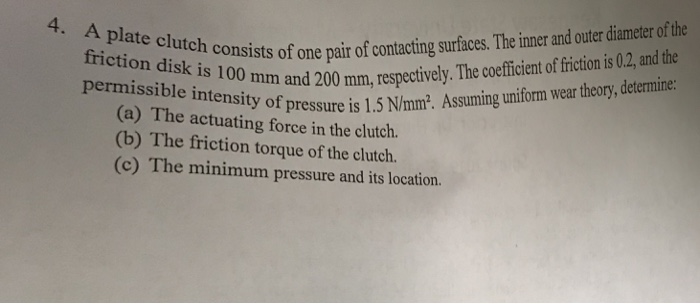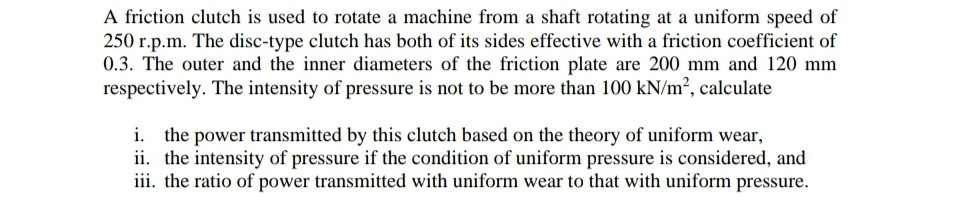Question

# Machine Layout

A plate clutch consists of 1 pair of contacting surfaces. The inner and outer diameter of the friction disk is 100mm and 200mm respectively. The coefficient of friction is 0.2 and permissible intensity of pressure is 1.5N/mm². Assuming uniform wear theory, calculate the operating force in the clutch.
a) 15546N
b) 12344N
c) 23562N
d) 24543N

Explanation: P=πpd(D-d)/2.

#### Earn Coins

Coins can be redeemed for fabulous gifts.

Similar Homework Help Questions
• ### Machine Layout

A plate clutch consists of 1 pair of contacting surfaces. The inner and outer diameter of the friction disk is 100mm and 200mm respectively. The coefficient of friction is 0.2 and permissible intensity of pressure is 1.5N/mm². Assuming uniform wear theory, calculate the power transmitting capacity of the clutch at 80rad/s.a) 27.8kWb) 32.4kWc) 21.2kWd) 34.5kW

• ### Machine Layout

A plate clutch consists of 1 pair of contacting surfaces. The inner and outer diameter of the friction disk is 100mm and 200mm respectively. The coefficient of friction is 0.2 and permissible intensity of pressure is 1.5N/mm². Assuming uniform wear theory, calculate the power transmitting capacity of the clutch at 80rad/s.a) 27kWb) 32kWc) 39kWd) 44kW

• ### Machine Layout

A plate clutch consists of 1 pair of contacting surfaces. The inner and outer diameter of the friction disk is 100mm and 200mm respectively. The coefficient of friction is 0.2 and permissible intensity of pressure is 1.5N/mm². Assuming uniform wear theory, calculate the torque transmitting capacity of the clutch.a) 412.23N-mb) 353.43N-mc) 334.53N-md) 398.34N-m

• ### Machine Layout

A plate clutch consists of 1 pair of contacting surfaces. The inner and outer diameter of the friction disk is 100mm and 200mm respectively. The coefficient of friction is 0.2 and permissible intensity of pressure is 1.5N/mm². Assuming uniform pressure theory, calculate the operating force in the clutch.a) 15546Nb) 12344Nc) 23562Nd) 35343N

• ### Machine Layout

A plate clutch consists of 1 pair of contacting surfaces. The inner and outer diameter of the friction disk is 100mm and 200mm respectively. The coefficient of friction is 0.2 and permissible intensity of pressure is 1.5N/mm². Assuming uniform pressure theory, calculate the torque transmitting capacity of the clutch.a) 412.23N-mb) 549.78N-mc) 567.54N-md) 678.86N-m

• ### Machine Layout

Find number of contacting surfaces for a multi disk clutch plate transmitting torque of 10N-m and inner and outer diameters of friction lining are 70mm and 100mm respectively. The operating force is of magnitude 305N and coefficient of friction is 0.2.a) 5b) 2c) 4d) 3

• ### Machine Layout

A cone clutch transmits 24kW at 490rpm. The coefficient of friction is 0.2 and allowable intensity of pressure is 0.35N/mm². The semi cone angle is 12⁰. The outer diameter is fixed as 310mm.Assuming uniform wear theory; find the inner diameter.a) 275mmb) 300mmc) 290mmd) 280mm

• ### fiction dist h consists of ome pair of contacting surfaces The ime and oer oth and...fiction dist h consists of ome pair of contacting surfaces The ime and oer oth and 200 mm, respecively. The coeficient of friction is 02, and the 4. A plate clutch consists of fri ction disk is 100 mm mm, resp sity of pressure is 1.5 N/mm2. Assuming uniform wear theory, determine: (a) The actuating force in the clutch. (b) The friction torque of the clutch. (c) The minimum pressure and its location.

• ### Machine Layout

A cone clutch transmits 24kW at 490rpm. The coefficient of friction is 0.2 and allowable intensity of pressure is 0.35N/mm². The semi cone angle is 12⁰. The outer diameter is fixed as 310mm.Assuming uniform wear theory; calculate force required to engage clutch.a) 3546.9Nb) 2231.5Nc) 3241.5Nd) 4354.5N

• ### A friction clutch is used to rotate a machine from a shaft rotating at a uniform...A friction clutch is used to rotate a machine from a shaft rotating at a uniform speed of 250 r.p.m. The disc-type clutch has both of its sides effective with a friction coefficient of 0.3. The outer and the inner diameters of the friction plate are 200 mm and 120 mm respectively. The intensity of pressure is not to be more than 100 kN/m², calculate i. the power transmitted by this clutch based on the theory of uniform wear, ii....